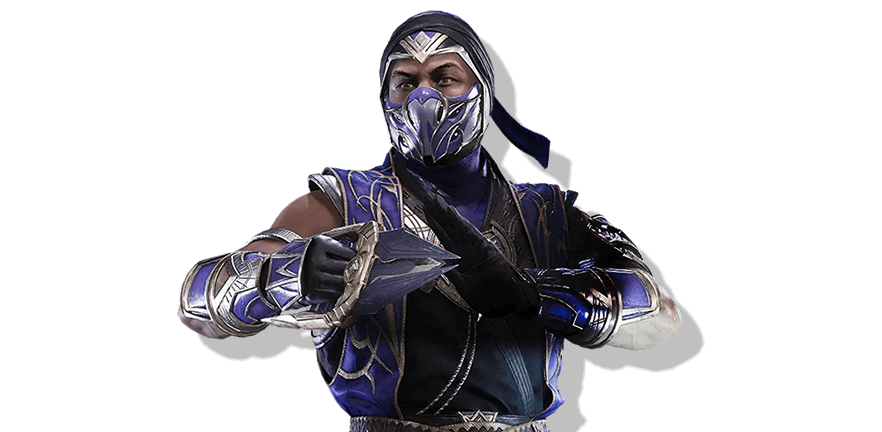Start learning movesets and combos!

Choose the platform you play on.
See how to read and perform inputs.Mortal Kombat 11

# RainCombos

The prince of Edenia, Rain is the adopted son of Argus, a powerful god in the Outworld.Know more

Get notified of the character’s updates

## Basic combo attacks

Combinations of basic moves

One
,
Three

One
,
Three
,
Three

One
,
Three
,
Four

Back
+
One
,
Four
or
Four
(hold)

Back
+
One
,
Four
hold
Back

Two
,
Four
,
Two

Two
,
Four
,
Two
,
One
+
Three

Two
,
Four
,
Down
+
Four

Back
+
Two
,
One

Back
+
Two
,
One
,
Two

Forward
+
Two
,
Down
+
Four

Forward
+
Two
,
One
+
Three

Forward
+
Two
,
One
+
Three
,
Four

### Rain and suffering

Back
+
Three
,
Four

Long combinations of various moves including abilities and specials

Down
+
Two
KB
Two
Four
Two
Down
Back
One

Down
+
Two
KB
Forward
+
Two
,
One
+
Three
,
Four

Back
+
Two
,
One
Back
Forward
Four
AMP
Forward
+
Two
,
One
+
Three
,
Four

Down
+
Two
KB
Two
Four
Two
,
One
+
Three

Back
+
Two
,
One
Back
Forward
Four
AMP
Two
Four
Two
Back
Forward
Three

Back
+
Two
,
One
Back
Forward
Four
AMP
Two
Four
Two
Down
Back
One

Forward
+
Two
,
One
+
Three
KBFB

Down
+
Two
KB
Two
Four
Two
Back
Forward
Four

Back
+
Two
,
One
Back
Forward
Four
AMP
Forward
+
Two
,
One
+
Three
,
Four

Forward
+
Two
,
One
+
Three
KBFB

Back
+
Two
,
One
Back
Forward
Four
AMP
Two
Four
Two
FB

Back
+
Two
,
One
Back
Forward
Four
AMP
Two
Four
Two
,
One
+
Three

One
Three
Three
Down
Back
One
AMP
Forward
Forward
Two
Four
Two
Down
Back
One

Down
+
Two
KB
Forward
+
Two
,
One
+
Three
,
Four

Back
+
Two
,
One
Back
Forward
Two
AMPhold
Down
Two
Four
Two
Back
Forward
Three

Back
+
Two
,
One
Back
Forward
Three
AMPKB
Forward
Forward
Two
Four
Two
,
One
+
Three

Down
+
Two
KB
Two
Four
Two
Back
Forward
Three

Back
+
Two
,
One
Back
Forward
Four
AMP
Two
Four
Two
FB

Back
+
Two
,
One
Back
Forward
Four
AMP
Two
Four
Two
FB